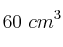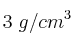# Material systems

Problems and exercises about the matter, properties of matter, density, the Kinetic Molecular Theory, changes os state, and the gases laws.

• (#4883)   Seleccionar

Mass of a volume of ethanol, expressed by pounds

The density of ethanol is 0.79 g/mL. Calculate the mass, expressed in pounds, of 17.4 mL of ethanol, knowing that one pound is equal to 0.45 kg. Express the result using scientific notation.

• (#4882)   Seleccionar

Will do float silver over mercury?

Imagine that you heat a system with upper temperatures than the fusion points of silver and mercury. If you put in contact both substances, will do silver float over mercury? Look for the information that you need on the Internet and explain your answer.

• (#4881)   Seleccionar

Volume occupied by a known mass of mercury

The density of mercury is 13.6 g/mL. What will be the volume of 25 000 g of mercury?

• (#4880)   Seleccionar

Mass of a piece of marble knowing its density and volume

What will be the mass of a piece of marble that occupiesvolume, if its density is?